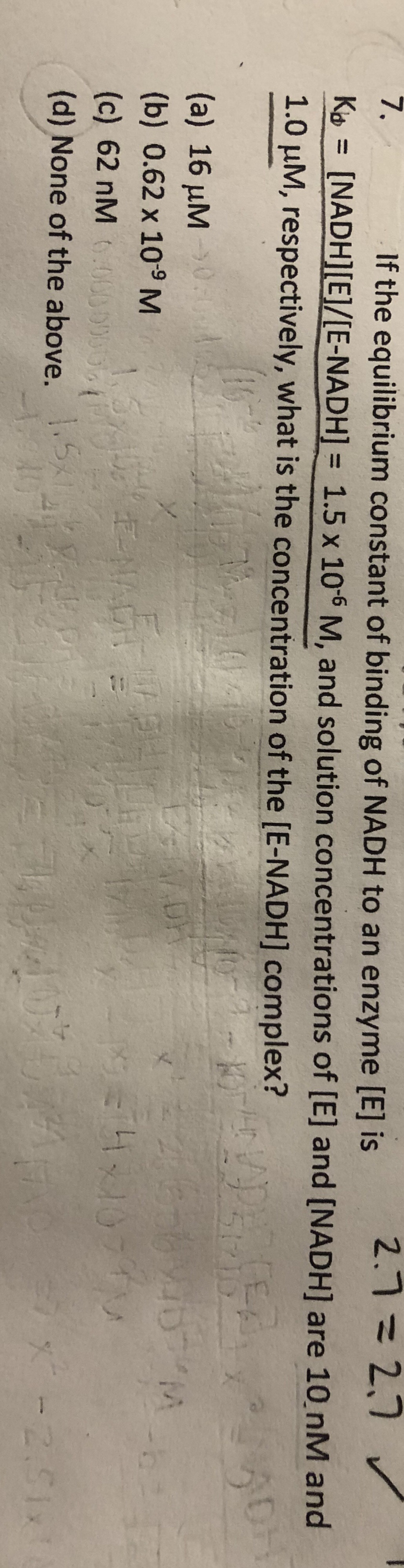# 7.If the equilibrium constant of binding of NADH to an enzyme [E] is2.7=2,7 vKo = [NADH][E]/[E-NADH] = 1.5 x 10-6 M, and solution concentrations of [E] and [NADH] are 10 nM and1.0 µM, respectively, what is the concentration of the [E-NADH] complex?%3D51210(a) 16 µM0.:0(b) 0.62 x 109 M1.5E-NADH(c) 62 nM .0000000(d) None of the above.5-2.51x

Question
42 viewshelp_outlineImage Transcriptionclose7. If the equilibrium constant of binding of NADH to an enzyme [E] is 2.7=2,7 v Ko = [NADH][E]/[E-NADH] = 1.5 x 10-6 M, and solution concentrations of [E] and [NADH] are 10 nM and 1.0 µM, respectively, what is the concentration of the [E-NADH] complex? %3D 51210 (a) 16 µM0.:0 (b) 0.62 x 109 M 1.5 E-NADH (c) 62 nM .0000000 (d) None of the above. 5 -2.51x fullscreen
check_circle

Step 1

The concentration of E is calculated in the unit of molar as follows:

Step 2

The concentration of NADH is calculated ...

### Want to see the full answer?

See Solution

#### Want to see this answer and more?

Solutions are written by subject experts who are available 24/7. Questions are typically answered within 1 hour.*

See Solution
*Response times may vary by subject and question.
Tagged in

### Chemistry